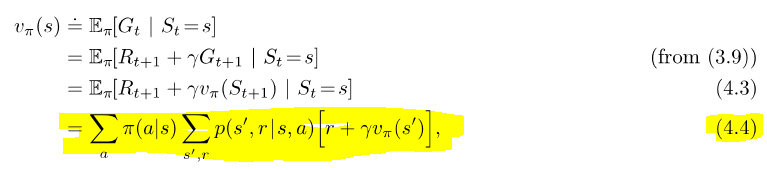The bellman expectation equation tells you the value for a given state (assuming a given policy). In other words, it tells you that if you start in a particular state, and follow a particular policy, you can expect this much return. So, it tells you how much return you can expect starting from each of your states and following the given policy.

The equation (taken from Sutton’s RL book):.

• given some policy, the value for state s is
• the expected return for following the policy starting at state s
• for each action, what is the probability of taking that action, what is the expected return for taking that action?
• “average” out all the actions
• the expected return for a particular action a is
• for each (subsequent_state, reward) pair that you might end up at
• probability that you’ll end up at that (subsequent_state, reward) * (reward + discount_factor * value(subsequent_state))

Iterative policy evaluation is an iterative algorithm for finding out the value function given a policy.

• arbitrarily initialize values for each state (they can be literally anything, i use all 0’s)
• while true
• for each state s
• calculate a new value by using bellman’s expectation equation
• termination condition: stop updating values when they stop changing by much